In: Chemistry

# SiF26− Number of Valence e- (Whole Structure) Areas of e- Density Around Central Atom: Electronic Geometry:...

SiF26

 Number of Valence e- (Whole Structure) Areas of e- Density Around Central Atom: Electronic Geometry:
 # Bonding Areas (Central Atom): # Non-Bonding Areas (Central Atom): Molecular Geometry
 Bond Angles (Central Atom)
 Polar Bonds?
 Polar Molecule?
 Any Pi (Double/Triple) Bonds?

## Solutions

##### Expert Solution

The given molecule is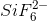.

Electronic configuration of Si =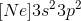Number of valence electron of Si = 4 e.

Electronic configuration of F=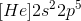Number of valence electrons = 7.

Since there are 6 F atoms, total valence electrons of F = 6*7 = 42 e.

There is a net charge of 2-, hence, that contributes extra 2 e.

Hence, Number of valence electrons of whole structure = 4+42+2 = 48 e.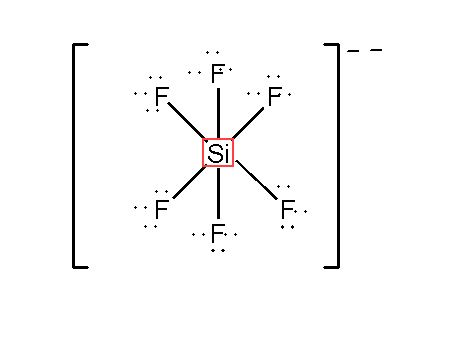Based on the Lewis structure,

# Areas of electron density around the central atom = 6

Electronic Geometry = Octahedral.

Si is forming sigma bond with each of the flourine atom.

Hence, # of bonding areas of central atom = 6.

# of non-bonding areas of central atom = 0.

Molecular geometry = Octahderal.

All the Si-F bond make 90 with each other about the central atom in octahedral geometry.

Bond angle (F-Si-F) = 90

Electronegativity of Si in Pauling's scale is about 1.9 whereas the same for F is about 4.

Hence, all the Si-F bonds are polar.

Polar Bonds? Yes, 6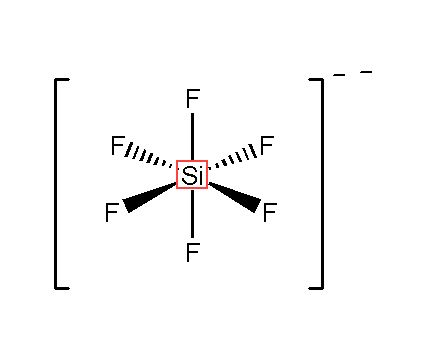Polar molecule? No

As the two Si-F bond dipoles on each spatial axis cancel the effect of the other as they are oriented opposite to each other. hence, the net dipole moment of the molecule is zero. making it non-polar.

Any Pi bond? No

Each F carry 6 electrons as lone pair, that is 36 e from 6 F. Remaining 12 electron are in the bond pairs, making the total 48. Hence, there is no more electron left to make any pi bonds.

## Related Solutions

##### 1. Give the number of lone pairs around the central atom and the molecular geometry of...
1. Give the number of lone pairs around the central atom and the molecular geometry of SeF4. Question 1 options: 0 lone pairs, tetrahedral 1 lone pair, distorted tetrahedron (seesaw) 1 lone pair, square pyramidal 1 lone pair, tetrahedral 2 lone pairs, square planar Save Question 2 Give the number of lone pairs around the central atom and the geometry of the ion SeO42–. Question 2 options: 0 lone pairs, square planar 1 lone pair, square pyramidal 1 lone pair,...
##### CH3F, SeO2, ICL4 Find Number of valence electrons, Molecular geometry, Hybridization of central atom, Polar and...
CH3F, SeO2, ICL4 Find Number of valence electrons, Molecular geometry, Hybridization of central atom, Polar and non Polar or ionic of them.
##### NOCl (N is the central atom) Draw the lewis structure Number of Valence electrons Formal charge
NOCl (N is the central atom) Draw the lewis structure Number of Valence electrons Formal charge
##### a) number of valence electrons, lewis structure, electron geometry, molecular geometry, bond angles, polar or non...
a) number of valence electrons, lewis structure, electron geometry, molecular geometry, bond angles, polar or non polar : ICl4^- B) lewis structure, electron geometry, molecular geometry, polar or non polar, sketch or molecule: IO3^1, SCN^-, BH3
##### Find point number of electron groups around central atom? Polarity? Number of Lone pairs for central...
Find point number of electron groups around central atom? Polarity? Number of Lone pairs for central atom? Lewis dot structure? Approximate bond angles? Electron pair arrangement around central atom/shape of the molecule? Hybridization of central atom? Symmetry elements and Points groups of compounds below. a) NF3 b) XeO3 c) SCl2
##### Predict the number of valence electrons in an atom of beryllium.
Predict the number of valence electrons in an atom of beryllium. Enter your answer in the provided box.
##### What is the molecular geometry around an atom in a molecule or ion which is surrounded...
What is the molecular geometry around an atom in a molecule or ion which is surrounded by one lone pair of electrons and four single bonds. Select one: a. linear b. T-shaped c. See-saw d. trigonal bipyramidal e. trigonal planar Which of the following concerning electron pair space requirements and bond angles is/are incorrect? 1. Lone pairs of electrons require more space than bonding pairs. 2. Multiple bonds require the same amount of space as single bonds. 3. The HOH...
##### The bond angles around the central atom in each structure are slightly different, however. The H-C-H...
The bond angles around the central atom in each structure are slightly different, however. The H-C-H bond angle in CH4 is 109.5., the H-O-H bond angle in H2O is about 104.5., and the H-O-H bond angle in H3O+ is approximately 107*. Explain these subtle differences in bond angles.
##### In the following molecules, predict the geometry, hybridization, and bond angle for the central atom. Give...
In the following molecules, predict the geometry, hybridization, and bond angle for the central atom. Give your answer using drawings. a) H3O                                                                                          b) BeCl2                                                          c) BCl3
##### What is the molecular geometry of a molecules whose central atom is sp3d hybridized and which...
What is the molecular geometry of a molecules whose central atom is sp3d hybridized and which consists of only bonding electrons?